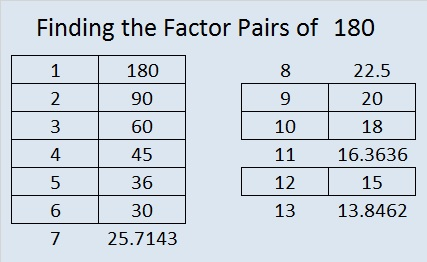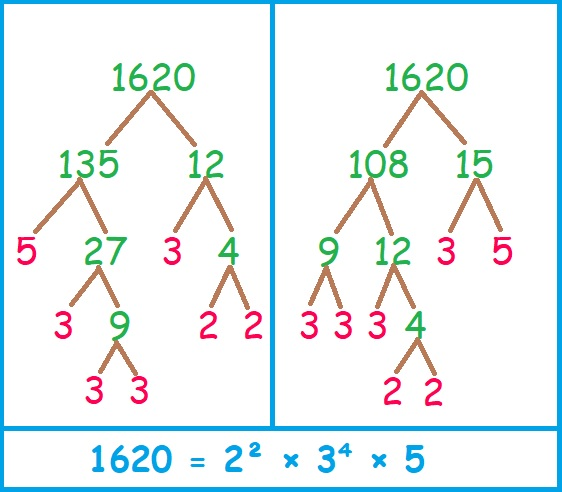# Math Happens When Factors of 1620 Make Sum-Difference

Contents

### Math Happens Puzzle:

Math Happens put another one of my puzzles in the Austin Chronicle and the Orange Leader!

You can see the puzzle on page 23 of this e-edition or this pdf of the newspaper. You can also find links to all of my Sum-Difference puzzles here.

Here is another one of Math Happen’s amazing puzzles. The tabletops shown are exactly the same. Click on it to see proof!

Math Happens in many different ways as you can see in their blog post from February 5. You can also look for Math Happens on a page in the middle of each of these  2020 issues or 2021 issues of the Austin Chronicle newspaper online.

Would you like puzzles like these in your community newspaper? Have your paper contact Math Happens on Twitter and make it happen!

### Today’s Sum-Difference Puzzles:

Just like the number 6 in the newspaper puzzle above, 180 and 1620 both have factor pairs that make sum-difference. To help you solve these puzzles, I’ve listed all of their factor pairs in the graphics below the puzzle.As shown below, 180 has nine factor pairs. One of those pairs adds up to 41, and another one subtracts to 41. Put the factors in the appropriate boxes in the first puzzle.The needed factors for the second puzzle are multiples of the numbers in the first puzzle. 1620 has fifteen factor pairs. One of the factor pairs adds up to ­123, and a different one subtracts to 123. If you can identify those factor pairs, then you can solve the second puzzle!

### What Are the Factors of 1620?• 1620 is a composite number.
• Prime factorization: 1620 = 2 × 2 × 3 × 3 × 3 × 3 × 5, which can be written 1620 = 2² × 3⁴ × 5.
• 1620 has at least one exponent greater than 1 in its prime factorization so √1620 can be simplified. Taking the factor pair from the factor pair table below with the largest square number factor, we get √1620 = (√324)(√5) = 18√5.
• The exponents in the prime factorization are 4, 2 and 1. Adding one to each exponent and multiplying we get (2 + 1)(4 + 1)(1 + 1) = 3 × 5 × 2 = 30. Therefore 1620 has exactly 30 factors.
• The factors of 1620 are outlined with their factor pair partners in the graphic above.

### Factor Trees for 1620:

Here are two of the MANY possible factor trees for 1620:Hint: I chose to build these factor trees with those two sets of factor pairs for a reason.

### More about the Number 1620:

1620 is the sum of the interior angles of a hendecagon (11-sided polygon) because
(11 – 2)180 = 1620.1620 is the sum of two squares:
36² + 18² = 1620.

1620 is the hypotenuse of a Pythagorean triple:
972-1296-1620, calculated from 36² – 18², 2(36)(18), 36² + 18².
It is also (3-4-5) times 324.

This is only some of the math that happens with the number 1620.

This site uses Akismet to reduce spam. Learn how your comment data is processed.